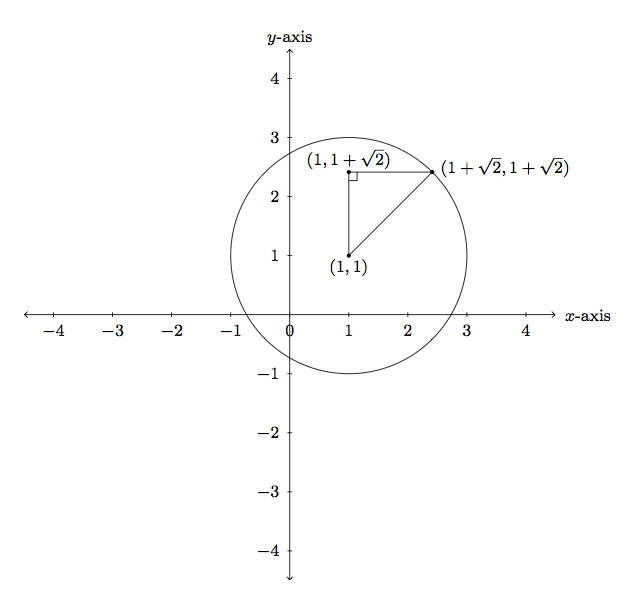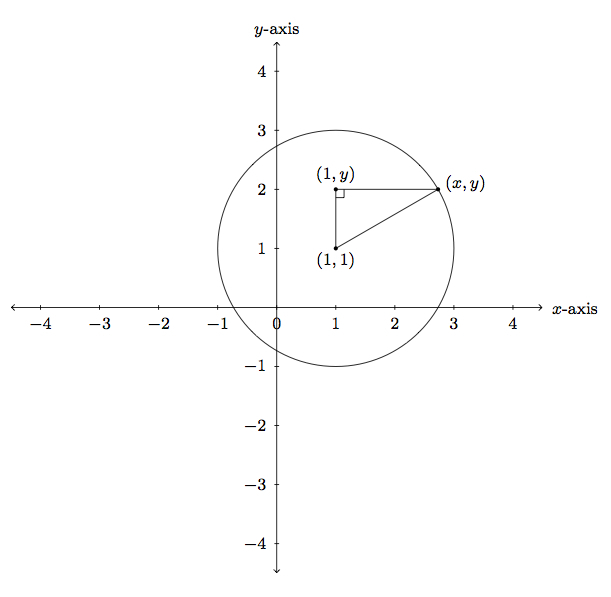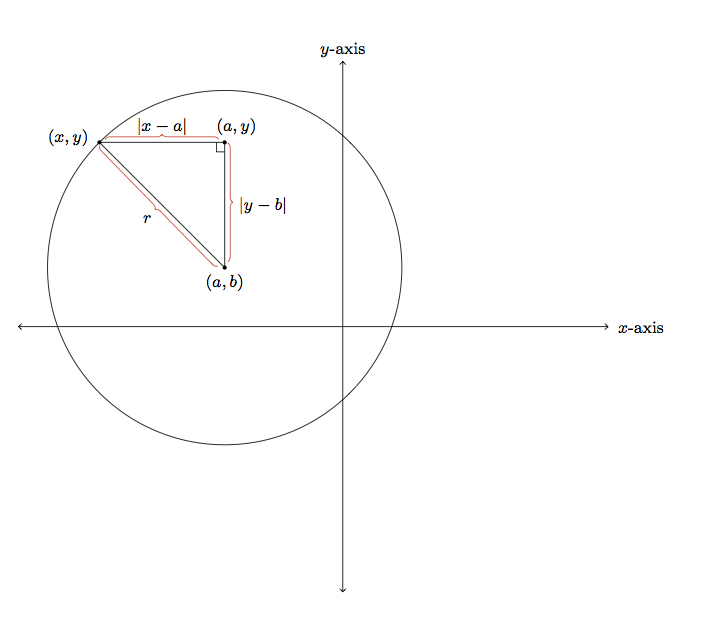Explaining the equation for a circle

Alignments to Content Standards: G-GPE.A.1

This problem examines equations defining different circles in the $x$-$y$ plane.

1. Use the Pythagorean theorem to find an equation in $x$ and $y$ whose solutions are the points on the circle of radius 2 with center $(1,1)$ and explain why it works.
2. Suppose $r$ is a positive number and $(a,b)$ a point in the plane. Use the Pythagorean theorem to find an equation in $x$ and $y$ whose solutions are the points on the circle of radius $r$ with center $(a,b)$ and explain why it works.

IM Commentary

Starting with explicit cases, this task derives the formula for an arbitrary circle in the plane. The teacher may wish to provide a hint, perhaps going over the first picture provided, and the accompanying reasoning, to help students visualize how the Pythagorean theorem can be applied to obtain information about points on the circle: if the teacher prefers an example with whole numbers, the radius could be changed to $5$ and, with center $(1,1)$, the point $(4,5)$ is on this circle. The equation $$(x-a)^2 + (y-b)^2 = r^2$$ for the circle of radius $r$ with center $(a,b)$ comes from applying the Pythagorean theorem to a carefully chosen right triangle with one vertex at $(a,b)$ and a second vertex on the circle. The pictures provided in the solution follow a progression from concrete to abstract: in the first picture for part (a), an explicit point is chosen on the explicit circle and the Pythagorean theorem is applied to obtain a relationship between the coordinates of the point on the circle and the coordinates of the circle center. This is then generalized by choosing an abstract point on the explicit circle. This abstract argument can be followed line for line in the general case considered in part (b), the only difference being that the coordinates of the center of the circle are now letters instead of numbers.

There are a couple of subtle points in this task which the teacher may wish to address:

• The natural logic of the argument shows that points $(x,y)$ lying on the circle of radius $r$ and center $(a,b)$ must satisfy the equation $(x-a)^2 + (y-b)^2 = r^2$. For logical completeness, the teacher may wish to address why only points on the circle satisfy this equation (this has been provided in the solution).
• Although the equation $(x-a)^2 + (y-b)^2 = r^2$ is the standard one for the circle of radius $r$ and center $(a,b)$ there are many others, for example $2(x-a)^2 + 2(y-b)^2 =2r^2$. In other words, there are infinitely many equations whose solutions define the same geometric object.

Successful work on this problem will require students to reason abstractly and quantitatively (MP2) in order to translate their geometric knowledge of what a circle is into an algebraic relationship. In addition they will need to attend to precision (MP6) once they have derived an equation as it is necessary to make sure that only the points on the circle satisfy this equation.

This task includes an experimental GeoGebra worksheet, with the intent that instructors might use it to more interactively demonstrate the relevant content material. The file should be considered a draft version, and feedback on it in the comment section is highly encouraged, both in terms of suggestions for improvement and for ideas on using it effectively. The file can be run via the free online application GeoGebra, or run locally if GeoGebra has been installed.

Attached Resources

• Geogebra File
• Solution

1. The circle of radius 2 with center $(1,1)$ is pictured below. To get a feel for how to apply the Pythagorean theorem in this setting, a point $(1 +\sqrt{2},1 + \sqrt{2})$ has been also been labelled on the circle:A third point $(1,1+\sqrt{2})$ has been added, making a right triangle along with the center of the circle and the point $(1+\sqrt{2},1+\sqrt{2})$. The Pythagorean theorem tells us that the sum of the squares of the legs of this triangle is equal to the square of the hypotenuse: $$(\sqrt{2})^2 + (\sqrt{2})^2 = 2^2.$$

More generally, if $(x,y)$ is any point on the circle of radius 2 centered at $(1,1)$ then this means that the distance from $(x,y)$ to $(1,1)$ is 2. A picture of this situation is given below:The lengths of the legs of the triangle are calculated as follows: for the horizontal the length is $|x-1|$ because the distance of two numbers on a line is found by taking the absolute value of their difference. Similarly, the vertical leg has length $|y-1|$. The hypotenuse has length 2 because $(x,y)$ lies on the circle of radius 2 and center $(1,1)$. By the Pythagorean theorem $$(x-1)^2 + (y-1)^2 = 2^2.$$ The absolute value signs are not necessary here because $(x-1)^2 = |x-1|^2$ and $(y-1)^2 = |y-1|^2$. So every point $(x,y)$ on the circle of radius $2$ with center $(1,1)$ satisfies the equation $$(x-1)^2 + (y-1)^2 = 2^2.$$

Conversely, with the exceptions of $(1,3), (1,-1), (3,1)$, and $(-1,1)$, every pair $(x,y)$ satisfying the equation $$(x-1)^2 + (y-1)^2 = 2^2$$ gives a triangle as above and a point on the circle with radius $2$ and center $(1,1)$: the Pythagorean theorem tells us that if $(x,y)$ satisfies this equation then it is a distance $2$ from $(1,1)$ and is therefore on the circle of radius $2$ and center $(1,1)$. The four exceptional cases noted above also lie on the circle of radius 2 with center $(1,1)$ but there is no triangle in this case, just a line segment of length 2.

2. Below is a picture of the center $(a,b)$ and a point $(x,y)$ on the circle of radius $r$ and center $(a,b)$:The lengths of the three sides are found as in part (a) and have been labelled: the hypotenuse is a radius of the circle and so has length $r$ and the lengths of the two legs are found by taking the absolute value of the differences of the $y$ coordinates for the vertical one and the $x$ coordinates for the horizontal leg. By the Pythagorean theorem $$(x-a)^2 + (y-b)^2 = r^2.$$ The absolute value signs are not necessary here because $(x-a)^2 = |x-a|^2$ and $(y-b)^2 = |y-b|^2$. So every point $(x,y)$ on the circle of radius $r$ with center $(a,b)$ satisfies the equation $$(x-a)^2 + (y-b)^2 = r^2.$$

Conversely, with the exceptions of $(a,b+r), (a,b-r), (a-r,b),$ and $(a+r,b)$, every pair $(x,y)$ satisfying the equation $$(x-a)^2 + (y-b)^2 = r^2$$ gives a triangle as above and a point on the circle with radius $r$ and center $(a,b)$: the Pythagorean theorem tells us that if $(x,y)$ satisfies this equation then it is a distance $r$ from $(a,b)$ and is therefore on the circle of radius $r$ and center $(a,b)$. The four exceptional cases noted above also lie on the circle of radius $r$ with center $(a,b)$ but there is no triangle in this case, just a line segment of length $r$.